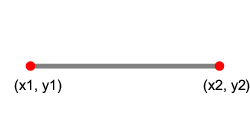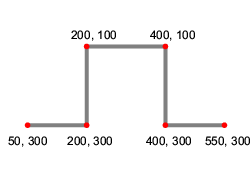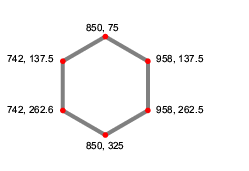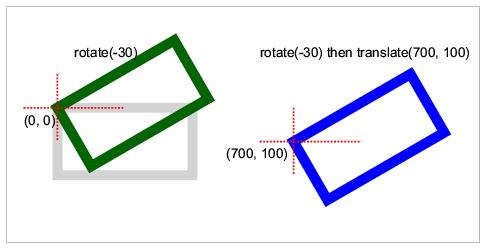# Scalable Vector Graphics (SVG)

SVG can be included in any XML document simply by adding a svg element.

The display, float and flow properties and all margin, padding, border and background properties can be applied to the top-level svg element but not to child elements within it.

The following elements are supported inside the svg element:

Element Usage
g for grouping related graphics elements
rect for defining rectangles
circle for defining circles
ellipse for defining ellipses
line for defining lines
polyline for defining polylines
polygon for defining polygons
path for defining arbitrary paths
text for defining text
tspan for adjusting text and font properties and the current text position inside the text element

## View box and view port

The svg element should include view box and view port information so that the SVG content can be correctly mapped to the containing box.

XML

```<svg width="3cm" height="2cm" viewBox="0 0 300 200">
<!-- the SVG content -->
</svg>
```
Attribute Meaning
width the width of the viewport
(100% of the container width, if omitted)
height the height of the viewport
(100% of the container width, if omitted)
viewBox
(x y width height)
the x, y coordinate of the top left corner of the view box and the width and height of the viewbox

## Rectangles

Rectangles are created using the rect element.

Attribute Meaning
x, y the x, y coordinate of the top left corner of the rectangle
width the width of the rectangle
height the height of the rectangle
rx, ry the radii for rounded corners

XML

```<svg viewBox="0 0 500 300"
width="10cm" height="6cm">
<g fill="none" stroke="gray" stroke-width="20">
<rect x="50" y="50" width="400" height="200"
rx="80" ry="50"/>
</g>
</svg>
```

Output## Circles

Circles are created using the circle element.

Attribute Meaning
cx, cy the centre of the circle
r the radius of the circle

XML

```<svg viewBox="0 0 200 200"
width="5cm" height="5cm">
<g fill="none" stroke="gray" stroke-width="20">
<circle cx="100" cy="100" r="100"/>
</g>
</svg>
```

Output## Ellipses

Ellipses are created using the ellipse element.

Attribute Meaning
cx, cy the centre of the ellipse
rx, ry the radii of the ellipse

XML

```<svg viewBox="-10 0 220 200"
width="6cm" height="6cm">
<g fill="none" stroke="gray" stroke-width="20">
<ellipse cx="100" cy="100" rx="100" ry="60" />
</g>
</svg>
```

Output## Lines

Lines are created using the line element.

Attribute Meaning
x1, y1 the start point of the line
x2, y2 the end point of the line

XML

```<svg viewBox="0 0 100 50"
width="6cm" height="3cm">
<g stroke="gray" stroke-width="2">
<line x1="10" y1="25" x2="90" y2="25"/>
</g>
</svg>
```

Output## Polylines

Polylines are created using the polyline element.

Attribute Meaning
points list of points that defines the line. Points are separated by space; each point is a pair of x, y coordinates separated by comma.

XML

```<svg viewBox="0 0 600 400"
width="6cm" height="4cm">
<g stroke="gray" stroke-width="10">
<polyline points="50,300 200,300 200,100
400,100 400,300 550,300" />
</g>
</svg>
```

Output## Polygons

Polygons are created using the polygon element.

Attribute Meaning
points list of points that defines the line. Points are separated by space; each point is a pair of x, y coordinates separated by comma.

XML

```<svg viewBox="600 0 600 400"
width="6cm" height="4cm">
<g fill="none" stroke="gray" stroke-width="10">
<polygon points="850,75  958,137.5 958,262.5
850,325 742,262.6 742,137.5" />
</g>
</svg>
```

Output## Paths

The path element is for defining arbitrary paths. The d attribute inside the element path takes a list of path commands. Parameters of a command can be separated either by a space or a comma.

Command Parameters Meaning
M (absolute)
m (relative)
(x y)+ moves to the given (x, y) coordinates to start a new subpath
Z or z none closes the current subpath
L (absolute)
l (relative)
(x y)+ draws a line to the given (x, y) coordinates
H (absolute)
h (relative)
x+ draws a horizontal line to the given x coordinates
V (absolute)
v (relative)
y+ draws a vertical line to the given y coordinates
C (absolute)
c (relative)
(x1 y1 x2 y2 x y)+ draws a cubic Bézier curve to the given (x,y) coordinates, uses the given (x1,y1) coordinates as the first control point, the (x2,y2) coordinates as the second control point
S (absolute)
s (relative)
(x2 y2 x y)+ short hand of the C/c command: the reflection relative to the current point of the second control point of the previous command is used as the first control point.
Q (absolute)
q (relative)
(x1 y1 x y)+ draws a quadratic cubic Bézier curve to the given (x,y) coordinates, uses the given (x1,y1) coordinates as the control point.
T (absolute)
t (relative)
(x y)+ short hand of the Q/q command: the reflection relative to the current point of the control point of the previous command is used as the control point.
A (absolute)
a (relative)
(rx ry x-axis-rotation large-arc-flag sweep-flag x y)+ draws an elliptical arc to (x, y): the size and rotation of the ellipse are defined by two radii (rx, ry) and the x-axis-rotation; the center (cx, cy) of the ellipse is also determined by the large-arc-flag and sweep-flag constraint.

XML

```<svg viewBox="0 0 600 400"
width="12cm" height="8cm">
<g fill="none" stroke="red" stroke-width="10">
<path d="M100,200 C100,100 250,100
250,200 S400,300 400,200" />
</g>
</svg>
```

Output## Text

The text element is supported inside the svg element; the tspan element is supported inside the text element.

Attribute Meaning
x, y the absolute position of the text
dx, dy the relative offset of the current text position (optional)

XML

```<svg viewBox="0 0 1000 300"
width="10cm" height="3cm">
<text x="200" y="150" fill="blue" font-size="70">
That
<tspan dx="2em" dy="-50" font-weight="bold" fill="red" >
is
</tspan>
<tspan dy="100">
a peach!
</tspan>
</text>
</svg>
```

Output## Transformations

The transform attribute can be used on g, path and all of the basic shape elements. It accepts any sequence of the following transformations separated by spaces.

• translate(tx ty)
• scale(sx [sy])
• rotate(angle)
• skewX(angle)
• skewY(angle)
• matrix(a b c d e f)

XML

```<svg viewBox="-150 -200 1400 700" width="12cm" height="6cm">
<g fill="none" stroke-width="30">
<rect width="400" height="200" stroke="lightgray"/>
<rect width="400" height="200" stroke="darkgreen" transform="rotate(-30)"/>
<g transform="translate(700 100) rotate(-30)">
<rect width="400" height="200" stroke="blue"/>
</g>
</g>
</svg>
```

Output## Style Properties

The following style properties are supported and can be applied to SVG elements:

• font-family
• font-size
• font-style
• font-weight
• fill
• fill-rule
• stroke
• stroke-linecap
• stroke-linejoin
• stroke-dasharray
• stroke-dashoffset
• stroke-width
• text-anchor

There are two ways to apply style properties:

• Using presentation attributes:
```<rect fill="yellow" stroke="blue" stroke-width="20"
width="200" height="100"/>
```
• Using the style attribute:
```<rect
style="fill:yellow; stroke:blue; stroke-width:20"
width="200" height="100"/>
```
the value of the style attribute is a list of style properties separated by semi-colons; each property is a name-value pair separated by a colon.